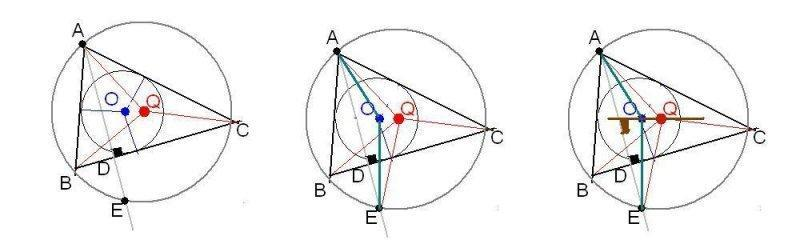# Incircle trig problem

## Homework Statement

ABC is a triangle in which none of the angles is obtuse. The perpendicular AD from A to BC is produced to meet the circumcircle of the triangle at E. If D is equidistant from A and E prove that the triangle must be right-angled. If, alternatively, the incentre of the triangle is equidistant from A and E, prove that cos B + cos C = 1.

## The Attempt at a Solution

I can see that D must lie on the diameter as must B and C, so A must be 90 degrees. The second part of the question is where I am stumped. I have been trying to find an expression that constrains the incircle to be equidistant from A and E and transform that expression to the required one. I toyed with r = (a+b+c) where r is the radius of the incircle and a, b, c are sides of the triangle. However, I couldn't make any progress.

Just noticed that the r = a+b+c is gibberish. Please ignore this.

Not sure how to interepret the lack of response on this; uninteresting problem?difficult problem? Breach of forum etiquette? I posted on a few other forums with a similarly limited response. It's one of the last questions in my text book, shame to fail so tantalisingly close to the end, so if anyone could help me reach that fulfilling sense of closure that a correct answer might provide I'd be very pleased.

BvU
Homework Helper
Sympathize, but can't offer any useful assitance, sorry. (For me they are difficult exercises -- did draw some triangles and circles, but couldn't find a route to go)

Been watching this thread, will be watching the trig identity one too. Perhaps @ehild ?

SammyS
Staff Emeritus
Homework Helper
Gold Member
Not sure how to interepret the lack of response on this; uninteresting problem?difficult problem? Breach of forum etiquette? I posted on a few other forums with a similarly limited response. It's one of the last questions in my text book, shame to fail so tantalisingly close to the end, so if anyone could help me reach that fulfilling sense of closure that a correct answer might provide I'd be very pleased.
If you would include a diagram or sketch, that might help.

ehild
Homework Helper
If you would include a diagram or sketch, that might help.

I attach a picture for the second question (the first is solved by the OP) .O is the centre of the incircle with radius r, Q is the centre of the circumcircle of the triangle and the quadrilateral ABEC. As AO = OE and AQ = QE both O and Q are on the bisector of AE, and the line OQ is parallel to the side BC of the triangle, so both are at distance r from the side BC. Using that the incentre is the cross section of angle bisectors and the centre of circumcircle is the cross section of side bisectors, there are some useful right triangles. If a is the side BC, I got the equations a=r(ctg(B/2)+ctg(C/2)) and a=2 r tan(A), but haven't succeeded to go much further.

ehild
Homework Helper
I succeeded at last. :)
Eliminating r from a=r(ctg(B/2)+ctg(C/2)) and a=2 r tan(A), and using that tan(A)=-tan(B+C),
##\frac{\cos(B/2}{\sin(B/2)}+\frac{\cos(C/2}{\sin(C/2)}=-2\frac{\sin(B+C)}{\cos(B+C)}##
Bringing the LHS to common denominator, using the addition formula sin(x+y)=sin(x)cos(y)+cos(x)sin(y), the equation can be simplified with sin((B+C)/2).
##\frac{1}{\sin(B/2)sin(C/2)}=-4 \frac{\cos((B+C)/2)}{\cos(B+C)}##

Using the identities sin(x)sin(y)=0.5(cos(x-y)-cos(x+y)), cos(x)cos(y)=0.5(cos(x+y)+cos(x-y)), and cos2(x/2)=0.5(1+cos(x)), we arrive at the desired formula.
I think there must be a simpler solution!

•BvU